## Quantities for Comparison

According to our preliminary calculations, self induced periodic oscillations develop in the flow and structure. The comparison will be done for fully developed flow, and particularly for one full period of the oscillation with respect to the position of the point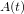$A(t)$. The suggested quantities of interest are:

• The y-coordinate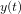$y(t)$ of the end of the beam structure at point$A(t)$ (see Figure 2).
• Forces exerted by the fluid on the whole submerged body, i.e. lift and drag forces acting on the cylinder and the beam structure together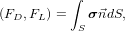$\begin{displaymath}(F_D,F_L)= \int_S \tens{\sigma} \vec{n} dS,\end{displaymath}$
where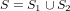$S=S_1 \cup S_2$ (see Figure) denotes the part of the circle being in contact with the fluid (i.e.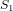$S_1$) plus part of the boundary of the beam structure being in contact with the fluid (i.e.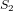$S_2$), and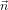$\vec{n}$ is the outer unit normal vector to the integration path with respect to the fluid domain.

The forces can be calculated in several different ways, i.e.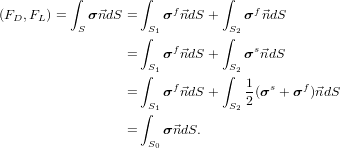\begin{align*} (F_D,F_L)= \int_S \tens{\sigma} \vec{n} dS =& \int_{S_1} \tens{\sigma}^f \vec{n} dS + \int_{S_2} \tens{\sigma}^f \vec{n} dS \\ =& \int_{S_1} \tens{\sigma}^f \vec{n} dS + \int_{S_2} \tens{\sigma}^s \vec{n} dS \\ =& \int_{S_1} \tens{\sigma}^f \vec{n} dS + \int_{S_2} \frac12 ( \tens{\sigma}^s + \tens{\sigma}^f ) \vec{n} dS \\ =& \int_{S_0} \tens{\sigma} \vec{n} dS. \end{align*}

That means that, up to numerical effects, all proposed evaluations will lead (asymptotically) to the same results.

• Pressure difference between the points$A(t)$ and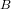$B$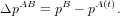$\begin{displaymath}\Delta p^{AB}=p^B-p^{A(t)}.\end{displaymath}$
The position of the point$A(t)$ is time dependent.The time dependent values are represented by the mean value, amplitude and frequency. The mean value and amplitude are computed from the last period of the oscillations by taking the maximum and minimum values, then the mean value is taken as average of the min/max values, and the amplitude is the difference of the max/min from the mean: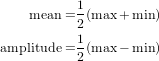\begin{align*} \text{mean}=& \frac12 (\max + \min) \\ \text{amplitude}=& \frac12 ( \max - \min) \end{align*}

The frequency of the oscillations can be computed either from the period time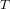$T$ as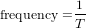\begin{align*} \text{frequency}=& \frac{1}{T} \end{align*}
or by using fourier analysis on the periodic data and taking the lowest significant frequency present in the spectrum. Additionally, a plot of the quantities over the period should be presented.